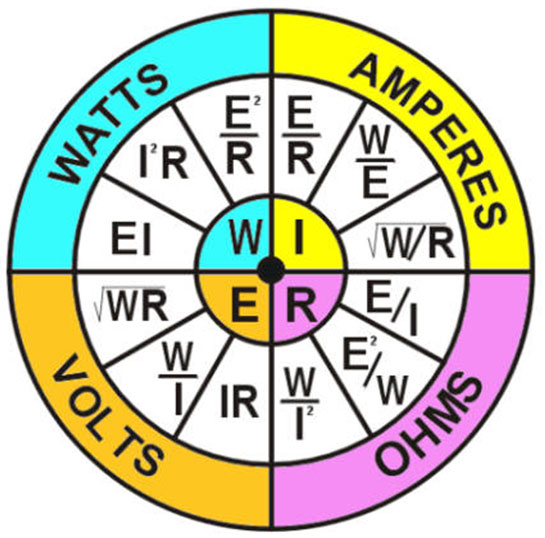# Ohm's Law

 E = Volts I = Current (Amperes) R = Resistance   I = E / R or Current = Voltage / Resistance E = I x R or Voltage = Current x Resistance R = V / E or Resistance = Voltage / CurrentKnowledge Centre File Search: Calculators